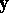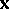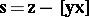# Rotation indicatrix

(diff) ← Older revision | Latest revision (diff) | Newer revision → (diff)
One of the twelve Darboux surfaces associated with the infinitesimal deformation of a surface. It is the set of points in space described by a position vectorwhich are parallel to the rotation vector (instantaneous angular velocity) defined by the equation, whereis the velocity vector of the infinitesimal deformation of the surface described by the position vector. The displacement indicatrix (displacement diagram) is defined in a similar manner by the displacement vector.